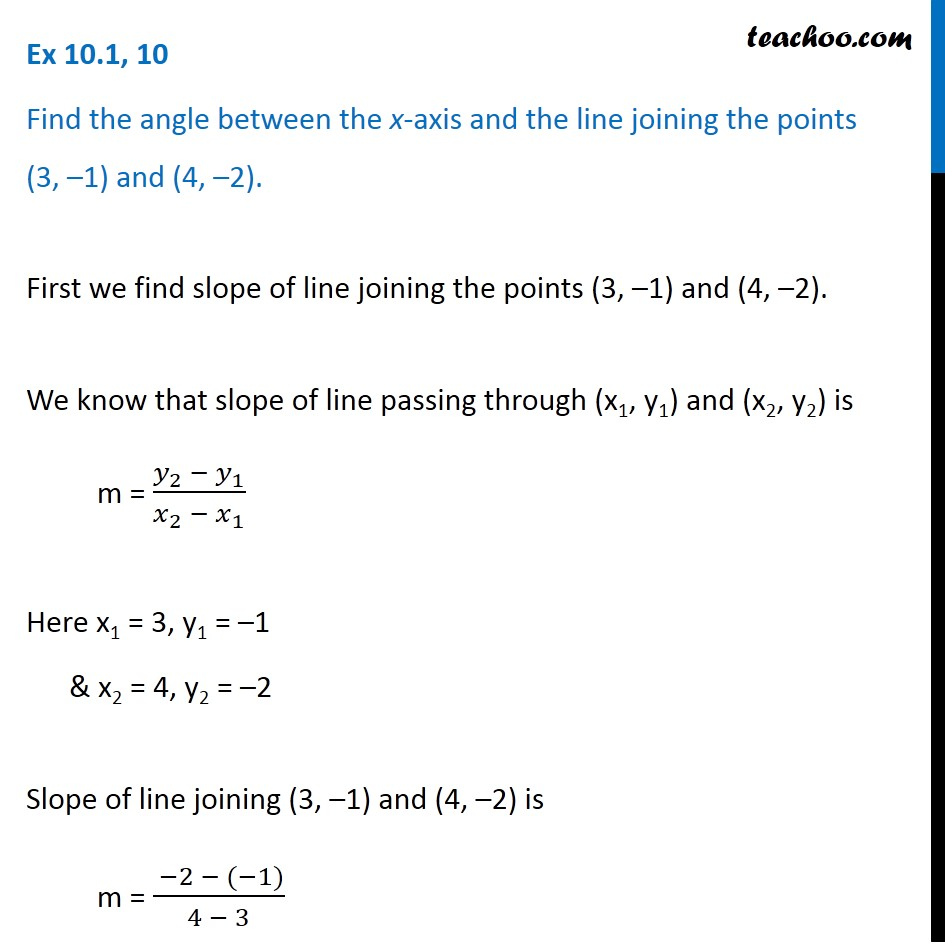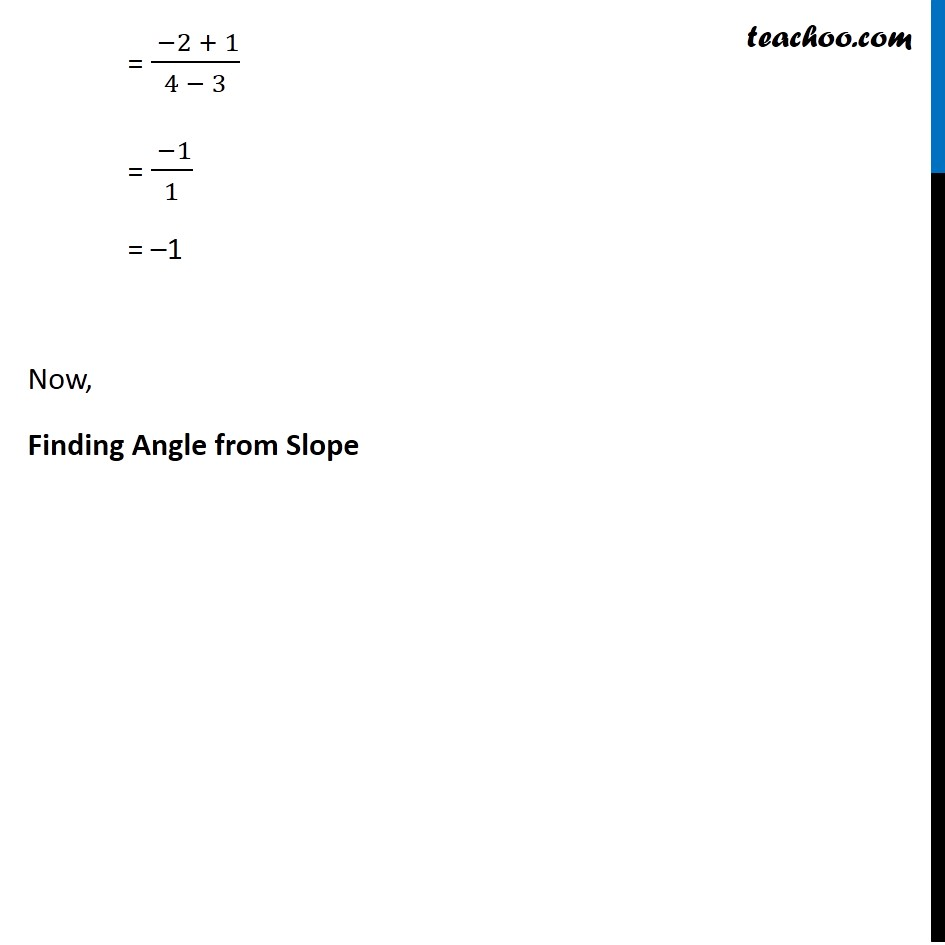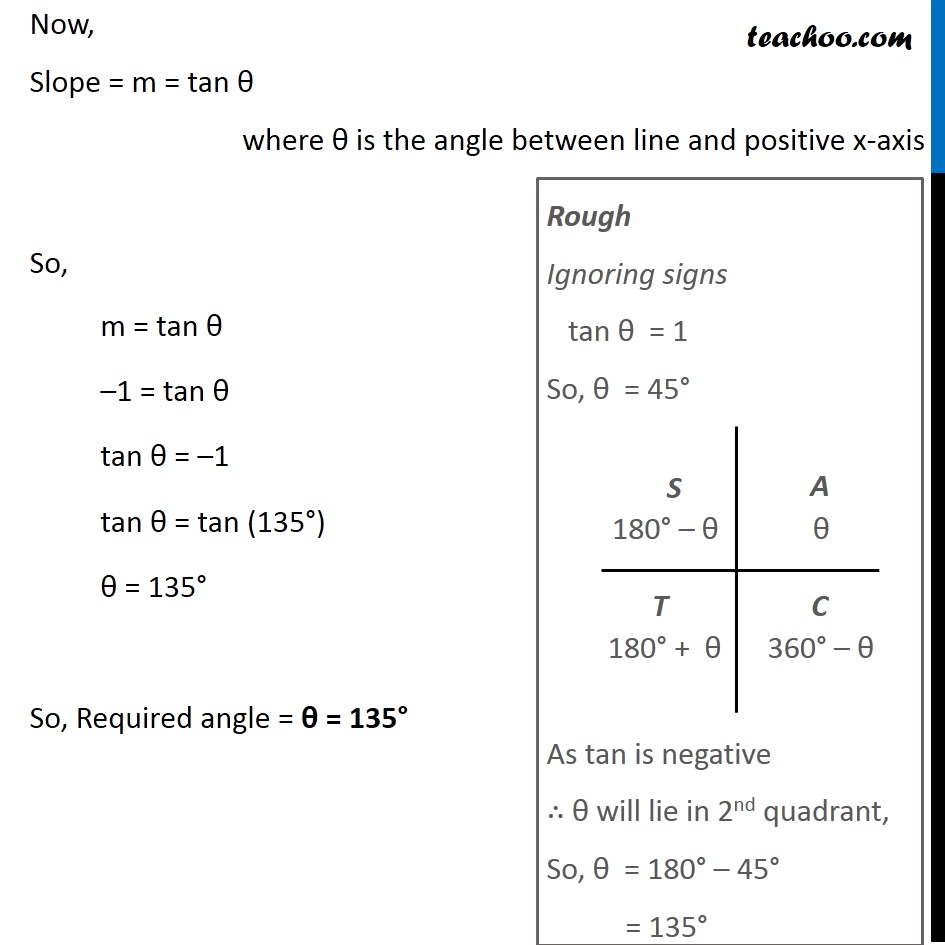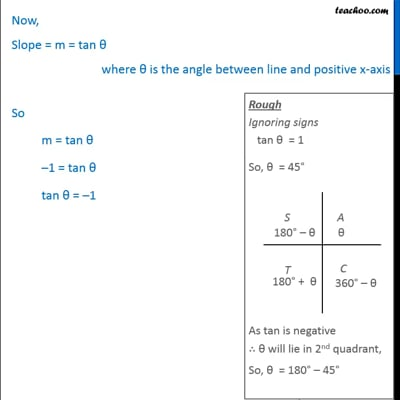Ex 10.1

Chapter 10 Class 11 Straight Lines (Term 1)
Serial order wiseThis video is only available for Teachoo black users

### Transcript

Ex 10.1, 10 Find the angle between the x-axis and the line joining the points (3, –1) and (4, –2). First we find slope of line joining the points (3, –1) and (4, –2). We know that slope of line passing through (x1, y1) and (x2, y2) is m = (𝑦_2 − 𝑦_1)/(𝑥_2 − 𝑥_1 ) Here x1 = 3, y1 = –1 & x2 = 4, y2 = –2 Slope of line joining (3, –1) and (4, –2) is m = ( −2 − (−1))/(4 − 3) = ( − 2 + 1)/(4 − 3) = ( − 1)/1 = –1 Ex 10.1, 10 Find the angle between the x-axis and the line joining the points (3, –1) and (4, –2). First we find slope of line joining the points (3, –1) and (4, –2). We know that slope of line passing through (x1, y1) and (x2, y2) is m = (𝑦_2 − 𝑦_1)/(𝑥_2 − 𝑥_1 ) Here x1 = 3, y1 = –1 & x2 = 4, y2 = –2 Slope of line joining (3, –1) and (4, –2) is m = ( −2 − (−1))/(4 − 3) = ( − 2 + 1)/(4 − 3) = ( − 1)/1 = –1 = ( −2 + 1)/(4 − 3) = ( −1)/1 = –1 Now, Finding Angle from Slope Now, Slope = m = tan θ where θ is the angle between line and positive x-axis So, m = tan θ –1 = tan θ tan θ = –1 tan θ = tan (135°) θ = 135° So, Required angle = θ = 135° Rough Ignoring signs tan θ = 1 So, θ = 45° As tan is negative ∴ θ will lie in 2nd quadrant, So, θ = 180° – 45° = 135°